JEE  >  Test: Single Correct MCQs: Electromagnetic Induction & Alternating Current | JEE Advanced

# Test: Single Correct MCQs: Electromagnetic Induction & Alternating Current | JEE Advanced

Test Description

## 16 Questions MCQ Test Physics For JEE | Test: Single Correct MCQs: Electromagnetic Induction & Alternating Current | JEE Advanced

Test: Single Correct MCQs: Electromagnetic Induction & Alternating Current | JEE Advanced for JEE 2023 is part of Physics For JEE preparation. The Test: Single Correct MCQs: Electromagnetic Induction & Alternating Current | JEE Advanced questions and answers have been prepared according to the JEE exam syllabus.The Test: Single Correct MCQs: Electromagnetic Induction & Alternating Current | JEE Advanced MCQs are made for JEE 2023 Exam. Find important definitions, questions, notes, meanings, examples, exercises, MCQs and online tests for Test: Single Correct MCQs: Electromagnetic Induction & Alternating Current | JEE Advanced below.
Solutions of Test: Single Correct MCQs: Electromagnetic Induction & Alternating Current | JEE Advanced questions in English are available as part of our Physics For JEE for JEE & Test: Single Correct MCQs: Electromagnetic Induction & Alternating Current | JEE Advanced solutions in Hindi for Physics For JEE course. Download more important topics, notes, lectures and mock test series for JEE Exam by signing up for free. Attempt Test: Single Correct MCQs: Electromagnetic Induction & Alternating Current | JEE Advanced | 16 questions in 30 minutes | Mock test for JEE preparation | Free important questions MCQ to study Physics For JEE for JEE Exam | Download free PDF with solutions
 1 Crore+ students have signed up on EduRev. Have you?
Test: Single Correct MCQs: Electromagnetic Induction & Alternating Current | JEE Advanced - Question 1

### A thin circular ring of area A is held perpendicular to a uniform magnetic field of induction B. A small cut is made in the ring and a galvanometer is connected across the ends such that the total resistance of the circuit is R. When the ring is suddenly squeezed to zero area, the charge flowing through the galvanometer is

Detailed Solution for Test: Single Correct MCQs: Electromagnetic Induction & Alternating Current | JEE Advanced - Question 1

The current induced will be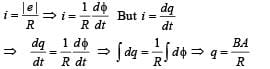Test: Single Correct MCQs: Electromagnetic Induction & Alternating Current | JEE Advanced - Question 2

### A thin semi-circular conducting ring of radius R is falling with its plane vertical in horizontal magnetic induction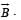At the position MNQ the speed of the ring is v, and the potential difference developed across the ring is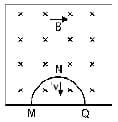Detailed Solution for Test: Single Correct MCQs: Electromagnetic Induction & Alternating Current | JEE Advanced - Question 2

Induced emf produced across MNQ will be same as the induced emf produced in straight wire MQ.

∴ e = Bvℓ = Bv × 2R with Q at higher potential.

Test: Single Correct MCQs: Electromagnetic Induction & Alternating Current | JEE Advanced - Question 3

### Two identical circular loops of metal wire are lying on a table without touching each other. Loop-A carries a current which increases with time. In response, the loop-B

Detailed Solution for Test: Single Correct MCQs: Electromagnetic Induction & Alternating Current | JEE Advanced - Question 3

When the current in the loop A increases, the magnetic lines of force in loop B also increases as loop A is placed near loop B. This induces an emf in B in such a direction that current flows opposite in B (as compared to A). Since currents are in opposite direction, the loop B  is repelled by loop A.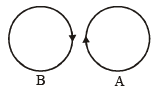Test: Single Correct MCQs: Electromagnetic Induction & Alternating Current | JEE Advanced - Question 4

A coil of inductance 8.4 mH and resistance 6 Ω is connected to a 12 V battery. The current in the coil is 1.0 A at approximately the time

Detailed Solution for Test: Single Correct MCQs: Electromagnetic Induction & Alternating Current | JEE Advanced - Question 4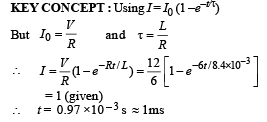Test: Single Correct MCQs: Electromagnetic Induction & Alternating Current | JEE Advanced - Question 5

A uniform but time-varying magnetic field B(t) exists in a circular region of radius a and is directed into the plane of the paper, as shown. The magnitude of the induced electric field at point P at a distance r from the centre of the circular region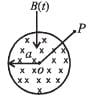Detailed Solution for Test: Single Correct MCQs: Electromagnetic Induction & Alternating Current | JEE Advanced - Question 5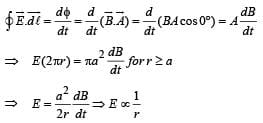Test: Single Correct MCQs: Electromagnetic Induction & Alternating Current | JEE Advanced - Question 6

A coil of wire having inductance and resistance has a conducting ring placed coaxially within it. The coil is connected to a battery at time t = 0, so that a time-dependent current l1(t) starts flowing through the coil. If I2(t) is the current induced in the ring, and B(t) is the magnetic field at the axis of the coil due to I1(t), then as a function of time (t > 0), the product I2(t) B(t)

Detailed Solution for Test: Single Correct MCQs: Electromagnetic Induction & Alternating Current | JEE Advanced - Question 6

KEY CONCEPT : The magnetic field at the centre of the coil

B(t) = µ0nI1.

As the current increases, B will also increase with time till it reaches a maximum value (when the current becomes steady).
The induced emf in the ring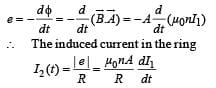[NOTE :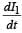decreases with time and hence I2 alsodecreases with time.] Where  I1 = Imax (1 – e –t/t)
The relevant graphs are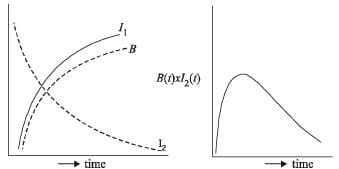Test: Single Correct MCQs: Electromagnetic Induction & Alternating Current | JEE Advanced - Question 7

A metallic square loop ABCD is moving in its own plane with velocity v in a uniform magnetic field perpendicular to its plane as shown in the figure. An electric field is induced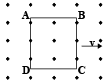Detailed Solution for Test: Single Correct MCQs: Electromagnetic Induction & Alternating Current | JEE Advanced - Question 7

NOTE : Electric field will be induced, as ABCD moves, in both AD and BC. The metallic square loop moves in its own plane with velocity v. A uniform magnetic field is imposed perpendicular to the plane of the square loop. AD and BC are perpendicular to the velocity as well as perpendicular to applied.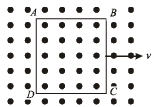Test: Single Correct MCQs: Electromagnetic Induction & Alternating Current | JEE Advanced - Question 8

Two circular coils can be arranged in any of the three situations shown in the figure. Their mutual inductance will be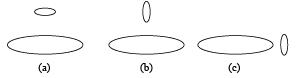Detailed Solution for Test: Single Correct MCQs: Electromagnetic Induction & Alternating Current | JEE Advanced - Question 8

Clearly the flux linkage is maximum in case (a) due to the spatial arrangement of the two loops.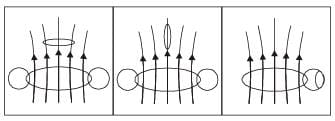Test: Single Correct MCQs: Electromagnetic Induction & Alternating Current | JEE Advanced - Question 9

As shown in the figure, P and Q are two coaxial conducting loops separated by some distance. When the switch S is closed, a clockwise current IP flows in P (as seen by E) and an induced current IQ1 flows in Q. The switch remains closed for a long time. When S is opened, a current IQ2 flows in Q.
Then the direction IQ1 and IQ2 (as seen by E) are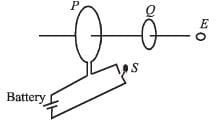Detailed Solution for Test: Single Correct MCQs: Electromagnetic Induction & Alternating Current | JEE Advanced - Question 9

When switch S is closed, a magnetic field is set-up in the space around P. The field lines threading Q increases in the direction from right to left. According to Lenz's law, IQ1 will flow so as to oppose the cause and flow in anticlockwise direction as seen by E.
Reverse is the case when S is opened. IQ2 will be clockwise.

Test: Single Correct MCQs: Electromagnetic Induction & Alternating Current | JEE Advanced - Question 10

A short-circuited coil is placed in a time-varying magnetic field. Electrical power is dissipated due to the current induced in the coil. If the number of turns were to be quadrupled and the wire radius halved, the electrical power dissipated would be

Detailed Solution for Test: Single Correct MCQs: Electromagnetic Induction & Alternating Current | JEE Advanced - Question 10

KEY CONCEPT :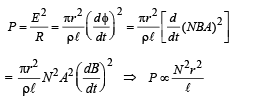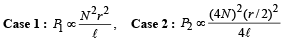NOTE : When we decrease the radius of the wire, its length increases but volume remains the same]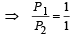∴ Power remains the same.

Test: Single Correct MCQs: Electromagnetic Induction & Alternating Current | JEE Advanced - Question 11

When an AC source of emf e = E0 sin(100t) is connected across a circuit, the phase difference between the emf e and the current i in the circuit is observed to be π/4 , as shown in the diagram. If the circuit consists possibly only of R-C or R-L or L-C in series, find the relationship between the two elements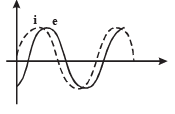Detailed Solution for Test: Single Correct MCQs: Electromagnetic Induction & Alternating Current | JEE Advanced - Question 11

NOTE :  Since current leads emf (as seen from the graph), therefore, this is an R – C circuit.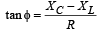Here φ = 45°

∴ XC = R [XL = 0 as there is no inductor]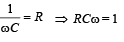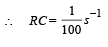Test: Single Correct MCQs: Electromagnetic Induction & Alternating Current | JEE Advanced - Question 12

A small bar magnet is being slowly inserted with constant velocity inside a solenoid as shown in figure. Which graph best represents the relationship between emf induced with time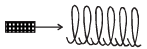Detailed Solution for Test: Single Correct MCQs: Electromagnetic Induction & Alternating Current | JEE Advanced - Question 12

KEY CONCEPT : Initially, φB increases as magnet approaches the solenoid

∴ ε = – ve and increasing in magnitude. When magnet is moving inside the solenoid, increase in φB slow down and finally φB starts decreasing

∴ emf is positive and increasing.
Only graph (c) shows these characteristic.

Test: Single Correct MCQs: Electromagnetic Induction & Alternating Current | JEE Advanced - Question 13

An infinitely long cylinder is kept parallel to an uniform magnetic field B directed along positive z-axis. The direction of induced current as seen from the z-axis will be

Detailed Solution for Test: Single Correct MCQs: Electromagnetic Induction & Alternating Current | JEE Advanced - Question 13

KEY CONCEPT : For a current to induce in cylindrical conducting rod,

(a) the conducting rod should cut magnetic lines of force which will happen only when the conducting rod is moving. Since conducting rod is  at rest, no current will be induced.
(b) the magnitude/direction of the magnetic field changes.
A changing magnetic field will create an electric field which can apply force on the free electrons of the conducting rod and a current will get induced.
But since the magnetic field is constant, no current will be induced.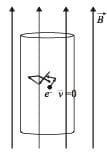Test: Single Correct MCQs: Electromagnetic Induction & Alternating Current | JEE Advanced - Question 14

Find the time constant (in μs) for the given RC circuits in the given order respectively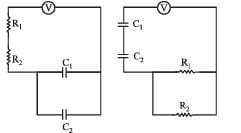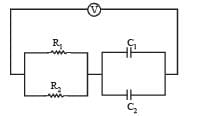R1 = 1W, R2 = 2W, C1 = 4μF , C2 =2μF

Detailed Solution for Test: Single Correct MCQs: Electromagnetic Induction & Alternating Current | JEE Advanced - Question 14

KEY CONCEPT :

Time constant of  R – C circuit is τ = Req Ceq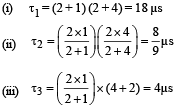Test: Single Correct MCQs: Electromagnetic Induction & Alternating Current | JEE Advanced - Question 15

The figure shows certain wire segments joined together to form a coplanar loop. The loop is placed in a perpendicular magnetic field in the direction going into the plane of the figure. The magnitude of the field increases with time. I1 and I2 are the currents in the segments ab and cd. Then,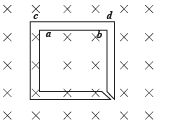Detailed Solution for Test: Single Correct MCQs: Electromagnetic Induction & Alternating Current | JEE Advanced - Question 15

The magnetic field is increasing in the downward direction. Therefore, according to Lenz’s law the current I1 will flow in the direction ab and I2 in the direction dc.

Test: Single Correct MCQs: Electromagnetic Induction & Alternating Current | JEE Advanced - Question 16

An AC voltage source of variable angular frequency ω and fixed amplitude V0 is connected in series with a capacitance C and an electric bulb of resistance R (inductance zero). When ω is increased

Detailed Solution for Test: Single Correct MCQs: Electromagnetic Induction & Alternating Current | JEE Advanced - Question 16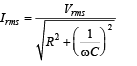As ω increases, Irms increases.

Therefore the bulb glows brighter

## Physics For JEE

258 videos|633 docs|256 tests
 Use Code STAYHOME200 and get INR 200 additional OFF Use Coupon Code
Information about Test: Single Correct MCQs: Electromagnetic Induction & Alternating Current | JEE Advanced Page
In this test you can find the Exam questions for Test: Single Correct MCQs: Electromagnetic Induction & Alternating Current | JEE Advanced solved & explained in the simplest way possible. Besides giving Questions and answers for Test: Single Correct MCQs: Electromagnetic Induction & Alternating Current | JEE Advanced, EduRev gives you an ample number of Online tests for practice

## Physics For JEE

258 videos|633 docs|256 tests